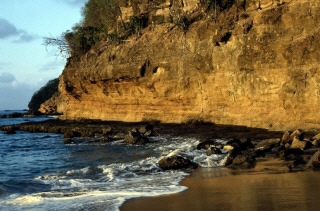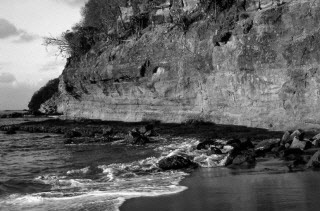←Select platform
In This Topic ▼

# GrayscaleCommand Class

Summary
Converts a 1-bit, 4-bit, 8-bit, 16-bit, 24-bit, or 32-bit image to an 8-bit, 12-bit, or 16-bit grayscale image.
Syntax
C#
Objective-C
C++/CLI
Java
Python
``public class GrayscaleCommand : RasterCommand ``
``@interface LTGrayscaleCommand : LTRasterCommand ``
``public class GrayscaleCommand extends RasterCommand ``
``public ref class GrayscaleCommand : public RasterCommand   ``
``class GrayscaleCommand(RasterCommand): ``
Remarks

Support for 12 and 16-bit grayscale images is available only in the Document/Medical Imaging editions.

The resulting image can be an 8-bit, 12-bit, or 16-bit grayscale image. Once the function is complete, the RasterImage.GrayscaleMode property will indicate the type of grayscale image.

When converting to 12-bit or 16-bit grayscale, the RasterImage.GetLookupTable is not used. When converting to 8-bit grayscale, the RasterImage.GetLookupTable is used to get the RGB for each input pixel. The grayscale value corresponding to that RGB triple is used in the destination image.

Grayscale 8-bit Function - BeforeGrayscale 8-bit Function - AfterView additional platform support for this Grayscale 8-bit function.

Example

This example will load a 24-bit image then convert it to a grayscale image with 8, 12 and 16 bits/pixel

C#
````using Leadtools; `
`using Leadtools.Codecs; `
`using Leadtools.ImageProcessing; `
` `
` `
`public void GrayscaleCommandExample() `
`{ `
`	RasterCodecs codecs = new RasterCodecs(); `
` `
`	string srcFileName = Path.Combine(LEAD_VARS.ImagesDir, "Image1.cmp"); `
`	string destFileName8 = Path.Combine(LEAD_VARS.ImagesDir, "Image1_grayscale8.jpg"); `
`	string destFileName12 = Path.Combine(LEAD_VARS.ImagesDir, "Image1_grayscale12.jpg"); `
`	string destFileName16 = Path.Combine(LEAD_VARS.ImagesDir, "Image1_grayscale16.jpg"); `
` `
`	// Load the source image from disk as 24-bits/pixel `
`	RasterImage image = codecs.Load(srcFileName, 24, CodecsLoadByteOrder.Bgr, 1, 1); `
` `
`	// Check the grayscale mode `
`	Console.WriteLine("Grsyscale mode of original image = {0}", image.GrayscaleMode); `
` `
`	// Convert to 8-bit grayscale `
`	GrayscaleCommand command = new GrayscaleCommand(); `
`	command.BitsPerPixel = 8; `
`	command.Run(image); `
` `
`	// Check the grayscale mode `
`	Console.WriteLine("Grsyscale mode after grayscale command with 8 bpp = {0}", image.GrayscaleMode); `
` `
`	// Save it to disk `
` `
`	codecs.Options.Jpeg.Save.QualityFactor = 2; `
`	codecs.Save(image, destFileName8, RasterImageFormat.Jpeg, 8); `
`	image.Dispose(); `
` `
`	// Load the image again this time grayscale to 12-bits/pixel `
`	image = codecs.Load(srcFileName, 24, CodecsLoadByteOrder.Bgr, 1, 1); `
`	command.BitsPerPixel = 12; `
`	command.Run(image); `
`	Console.WriteLine("Grsyscale mode after grayscale command with 12 bpp = {0}", image.GrayscaleMode); `
`	codecs.Options.Jpeg.Save.QualityFactor = 2; `
`	codecs.Save(image, destFileName12, RasterImageFormat.Jpeg, 12); `
`	image.Dispose(); `
` `
`	// Load the image again this time grayscale to 16-bits/pixel `
`	image = codecs.Load(srcFileName, 24, CodecsLoadByteOrder.Bgr, 1, 1); `
`	command.BitsPerPixel = 16; `
`	command.Run(image); `
`	Console.WriteLine("Grsyscale mode after grayscale command with 16 bpp = {0}", image.GrayscaleMode); `
` `
`	// When saving 16-bit jpegs, you must use lossless quality factor `
`	codecs.Options.Jpeg.Save.QualityFactor = 0; `
`	codecs.Save(image, destFileName16, RasterImageFormat.Jpeg, 16); `
`	image.Dispose(); `
` `
`	// Clean Up `
`	codecs.Dispose(); `
`} `
` `
`static class LEAD_VARS `
`{ `
`   public const string ImagesDir = @"C:\LEADTOOLS22\Resources\Images"; `
`} ````
Requirements